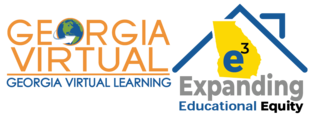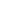GSE Geometry A SY 21-22 is a Course

GSE Geometry A SY 21-22

Started Jul 13, 2021

Full course description

GSE Geometry is the second course in a sequence of three required high school courses designed to ensure career and college readiness. The course represents a discrete study of geometry with correlated statistics applications. The focus of GSE Geometry is organized into 6 critical areas: 1. Building on standards from middle school, students will perform transformations in the coordinate plane, describe a sequence of transformations that will map one figure onto another, and describe transformations that will map a figure onto itself. Students will compare transformations that preserve distance and angle to those that do not. 2. Students will use transformations and proportional reasoning to develop a formal understanding of similarity and congruence. Students will identify criteria for similarity and congruence of triangles, develop facility with geometric proofs (variety of formats), and use the concepts of similarity and congruence to prove theorems involving lines, angles, triangles, and other polygons. 3. Students will apply similarity in right triangles to understand right triangle trigonometry. Students will use the Pythagorean Theorem and the relationship between the sine and cosine of complementary angles to solve problems involving right triangles. 4. Students will understand and apply theorems about circles, find arc lengths of circles, and find areas of sectors of circles. Students will develop and explain formulas related to circles and the volume of solid figures and use the formulas to solve problems. Building on standards from middle school, students will extend the study of identifying cross-sections of three-dimensional shapes to identifying three-dimensional objects generated by rotations of two-dimensional objects. 5. Students will use the concepts of distance, midpoint, and slope to verify algebraically geometric relationships of figures in the coordinate plane (triangles, quadrilaterals, and circles). Students will solve problems involving parallel and perpendicular lines, perimeters and areas of polygons, and the partitioning of a segment in a given ratio. Students will derive the equation of a circle and model real-world objects using geometric shapes and concepts. 6. Students will understand independence and conditional probability and use them to interpret data. Building on standards from middle school, students will formalize the rules of probability and use the rules to compute probabilities of compound events in a uniform probability model.